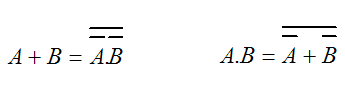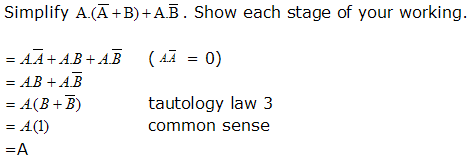# Computer ScienceBoolean Algebra

Look back through the last two pages. At every turn, we have been writing out expressions using boolean variables and the two symbols that express And & OR.

A few additional pieces of information will allow us to manipulate boolean expressions algebraically.

## De Morgan's Laws

You can look for a fuller explanation on T'Internet in your own time but, in their simplest form, these laws are,Look at the laws carefully. In each equation, the sign is changed from AND to OR or from OR to AND. The individual terms have a bar over them to indicate NOT. The whole of the expression has a bar over it. The following three steps describe this process succinctly,

1. NOT the sign
2. NOT the individual terms
3. NOT the lot

The implication for us is that, by substituting these values in boolean expressions, we can convert expressions to forms that require only the use of NOT & OR (NOR) functions or just NOT & AND (NAND) functions.

It is possible to make any logic circuit using only NOR or only NAND gates. Try it out for yourself. You should be able to create a circuit that results in the same output as any of the other logic gates - try it out for yourself. The advantage of this comes in manufacturing where costs can be kept down when only one type of gate is used.

## Some Other Rules To Bear In Mind

A + B = B + A
A.B = B.A

### Associative Laws

A + (B + C) = (A + B) + C
A.(B.C) = (A.B).C

### Distributive Laws

A.(B + C) = (A.B) + (A.C)
A + (B.C) = (A + B).(A + C)|

A.A = A
A + A = A

A + A = 1
A.A = 0

A = A

A + (A.B) = A
A.(A + B) = A

### Common Sense Laws

If you can't see why, draw a simple circuit with a power supply and lamp - you'll see.

0.A = 0
1 + A = 1
1.A = A
0 + A = A
0 = 1
1 = 0

## Example